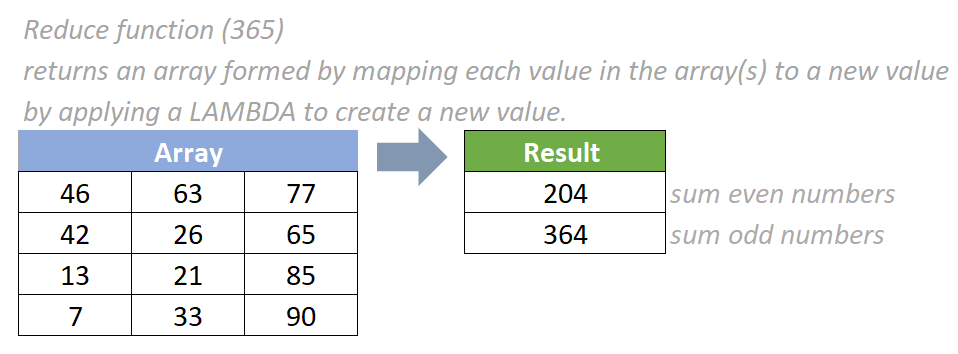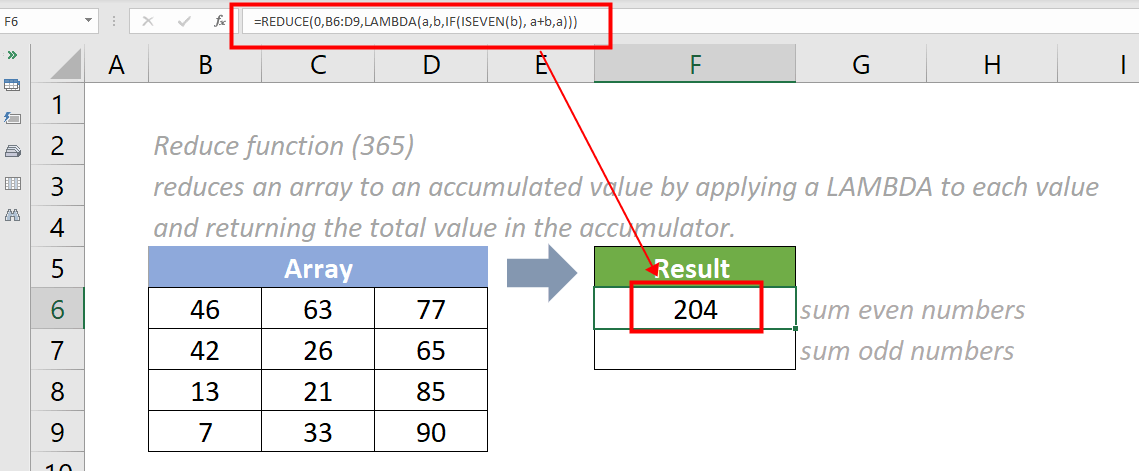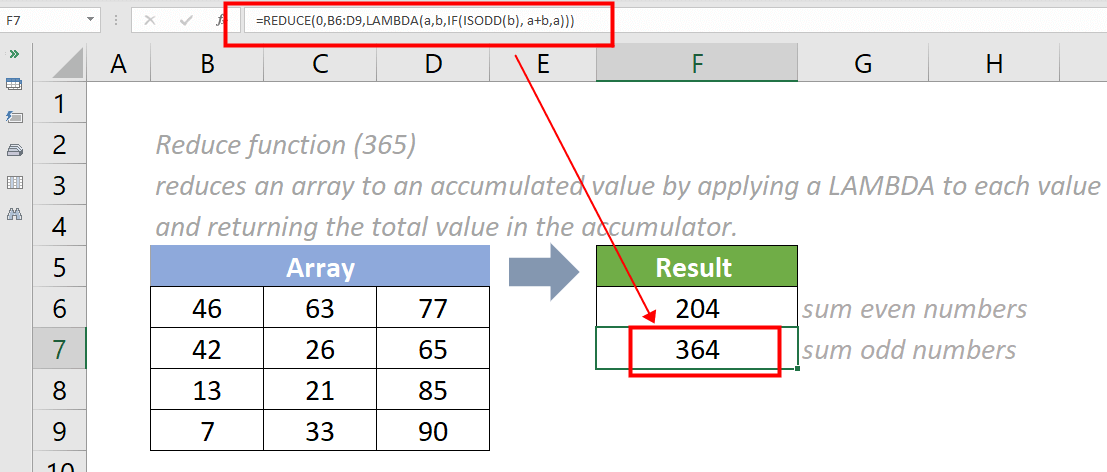## Excel REDUCE function (365)

The REDUCE function applies a LAMBDA function to each value in an array and returns the total value in the accumulator, reducing the array to an accumulated value.#### Syntax

=REDUCE ([initial_value],array,lambda(accumulator, value))

#### Arguments

• Initial_value (optional): The starting value of the accumulator. If omitted, the default value is 0.
• Array (required): The array to be reduced.
• Lambda (required): The custom LAMBDA function that is applied to reduce the array.
• Accumulator (required): The value totaled up and returned as the final result.
• Value (required): The calculation applied to each element in the array.

#### Return Value

The REDUCE function returns a single value.

#### Function notes

1. The REDUCE function is newly introduced in Excel for Microsoft 365. So it is not available in earlier versions of Excel.
2. The #VALUE! error value occurs if one of the below situations occur:
• an invalid LAMBDA function is provided;
• a wrong number of parameters is provided.

#### Example

As the below screenshot shows, there is an array of data. To sum the even and odd numbers in the array separately, please do as follows:

To sum the even numbers, please copy the formula below into cell F6, then press the Enter key to get the result.

=REDUCE (0,B6:D9,LAMBDA(a,b,IF(ISEVEN(b), a+b,a)))To sum the odd numbers, please copy the formula below into cell F7, then press the Enter key to get the result.

=REDUCE (0,B6:D9,LAMBDA(a,b,IF(ISODD(b), a+b,a)))Note: In each formula above, the supplied initial_value argument is set to 0. It can also be omitted and replaced by an empty space. For example, to sum the even numbers, the formula in cell F6 can be changed to:

=REDUCE ( ,B6:D9,LAMBDA(a,b,IF(ISEVEN(b), a+b,a)))

#### Relative Functions:

• Excel MAP Function
The MAP function applies a LAMBDA function to create a new value and returns an array formed by mapping each value in the supplied array(s) to a new value.

• Excel LAMBDA Function
The Excel LAMBDA function is used to create custom functions that can be reused throughout a workbook.

• Excel MAKEARRAY Function
The Excel MAKEARRAY function returns a calculated array based on the given number of rows and columns.